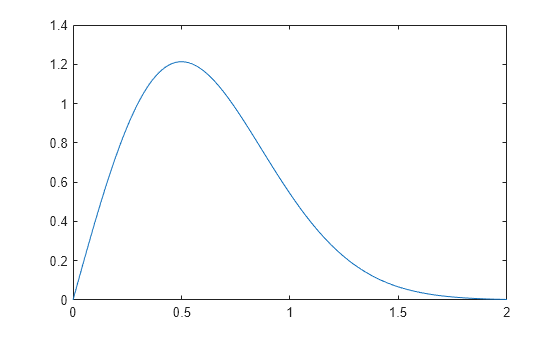## 瑞利分布

### 定义

`$y=f\left(x|b\right)=\frac{x}{{b}^{2}}{e}^{\left(\frac{-{x}^{2}}{2{b}^{2}}\right)}$`

### 参数

`raylfit` 函数返回瑞利参数的最大似然估计 (MLE)。此估计为

`$b=\sqrt{\frac{1}{2n}\sum _{i=1}^{n}{x}_{i}^{2}}$`

### 示例

#### 计算并绘制瑞利分布 pdf

```x = [0:0.01:2]; p = raylpdf(x,0.5);```

```figure; plot(x,p)```